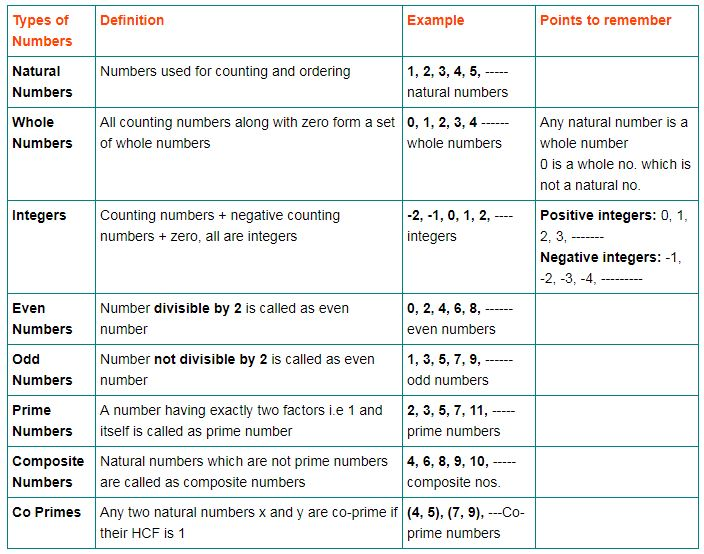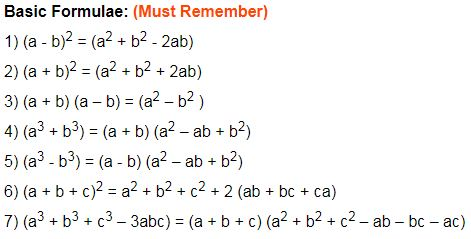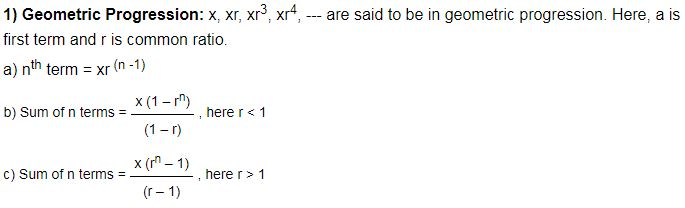# Numbers

#### Video Lesson on Numbers## Divisibility of Numbers

### Number divisible by 2

Units digit – 0, 2, 4, 6, 8

Example: 42, 66, 98, 1124

### Number divisible by 3

Sum of digits is divisible by 3

Example: 267 --- (2 + 6 + 7) = 15

15 is divisible by 3

### Number divisible by 4

Number formed by the last two digits is divisible by 4

Example: 832

The last two digits is divisible by 4, hence 832 is divisible by 4

### Number divisible by 5

Units digit is either zero or five

Example: 50, 20, 55, 65, etc

### Number divisible by 6

The number is divisible by both 2 and 3

Example: In 168 Last digit = 8 ---- (8 is divisible by 2)

Sum of digits = (1 + 6 + 8) = 15 ----- (divisible by 3)>/p>

Hence, 168 is divisible by 6

### Number divisible by 11

If the difference between the sums of the digits at even places and the sum of digits at odd places is either 0 or divisible by 11.

Example: 4527039

Digits on even places: 4 + 2 + 0 + 9 =15

Digits on odd places: 5 + 7 + 3 = 15

Difference between odd and even = 0

Therefore, number is divisible by 11

### Number divisible by 12

The number is divisible by both 4 and 3

Example: 1932

Last two digits divisible by 4

Sum of digits = (1 + 9 + 3 + 2) = 15 ---- (Divisible by 3)

Hence, the number 1932 is divisible by 12### Quick Tips and Tricks

If H.C.F of two numbers is 1, then the numbers are said to be co-prime. To find a number, say b is divisible by a, find two numbers m and n, such that m*n = a, where m and n are co-prime numbers and if b is divisible by both m and n then it is divisible by a.

Sum of the first n odd numbers = n2

Sum of first n even numbers = n ( n + 1)

Even numbers divisible by 2 can be expressed as 2n, n is an integer other than zero.

Odd numbers which are not divisible by 2 can be expressed as 2(n + 1), n is an integer.

Dividend = [(Divisor × Quotient)] + Remainder